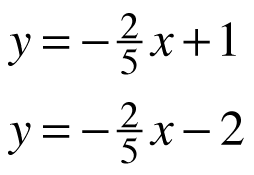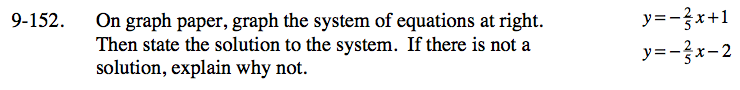### Home > CC3 > Chapter 9 > Lesson 9.2.7 > Problem9-152

9-152.
1. On graph paper, graph the system of equations below. Then state the solution to the system. If there is not a solution, explain why not. 9-152 HW eTool (Desmos). Homework Help ✎

2.Why is there no solution? How can you tell by looking at the equations?

Use the eTool below to graph the equations.
Click the link at right for the full version of the eTool: CC3 9-152 HW eTool